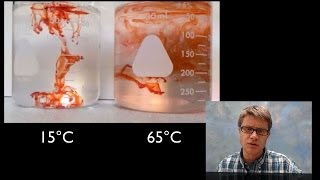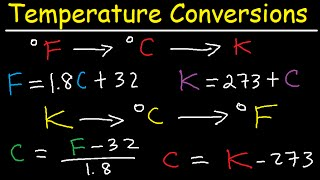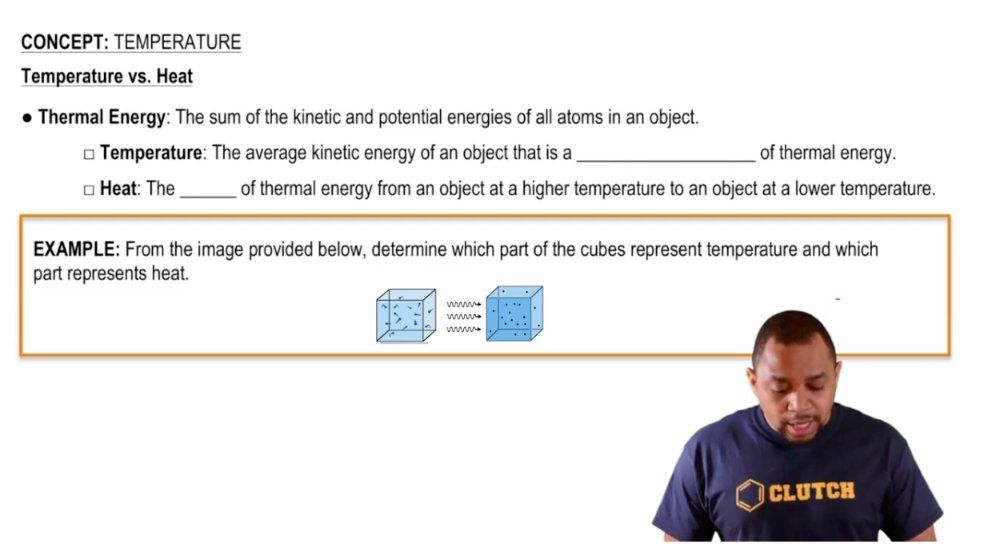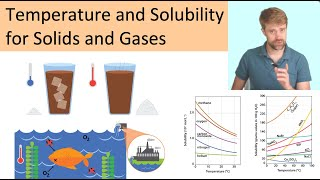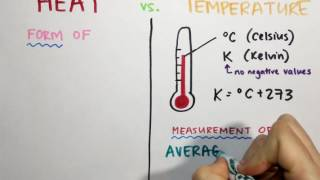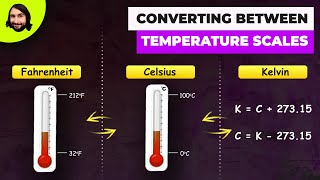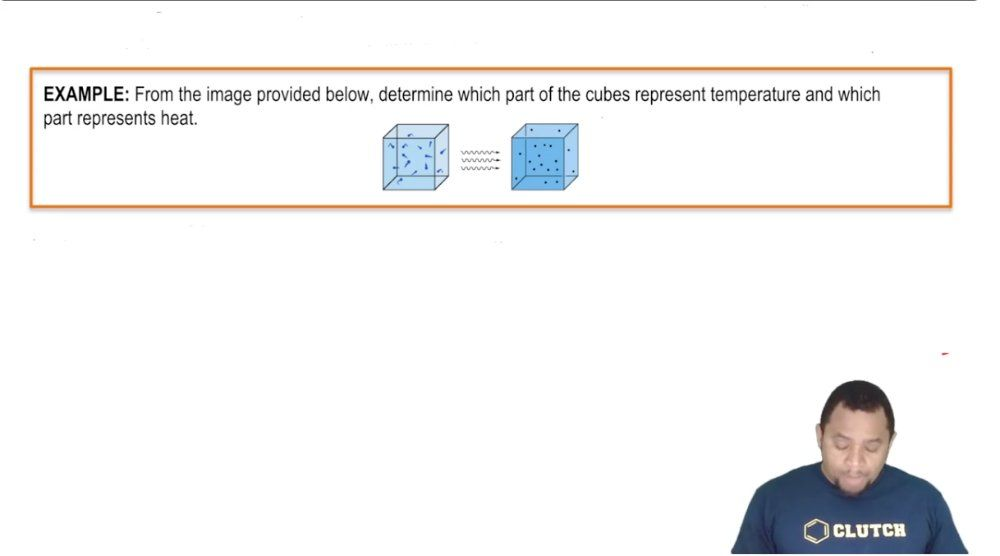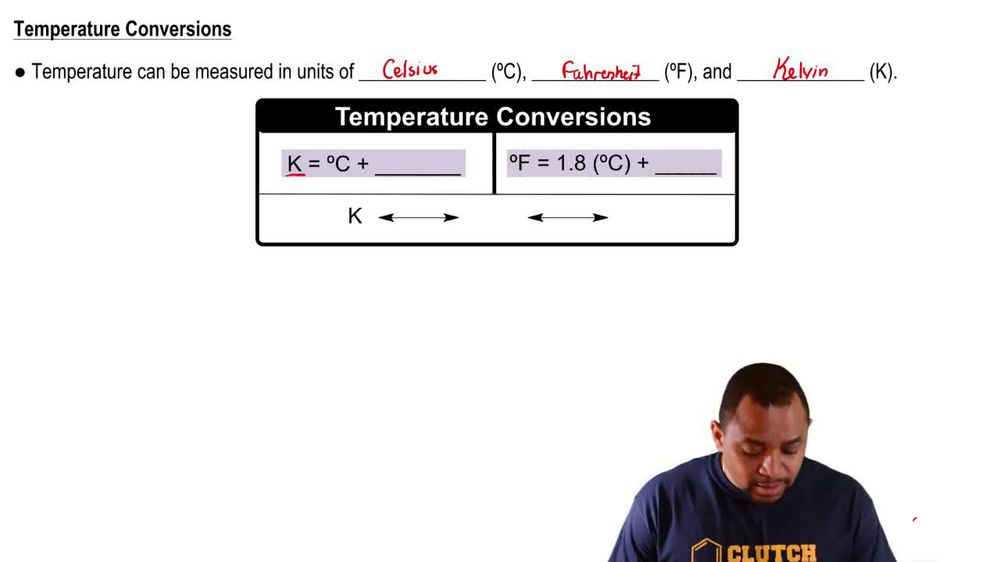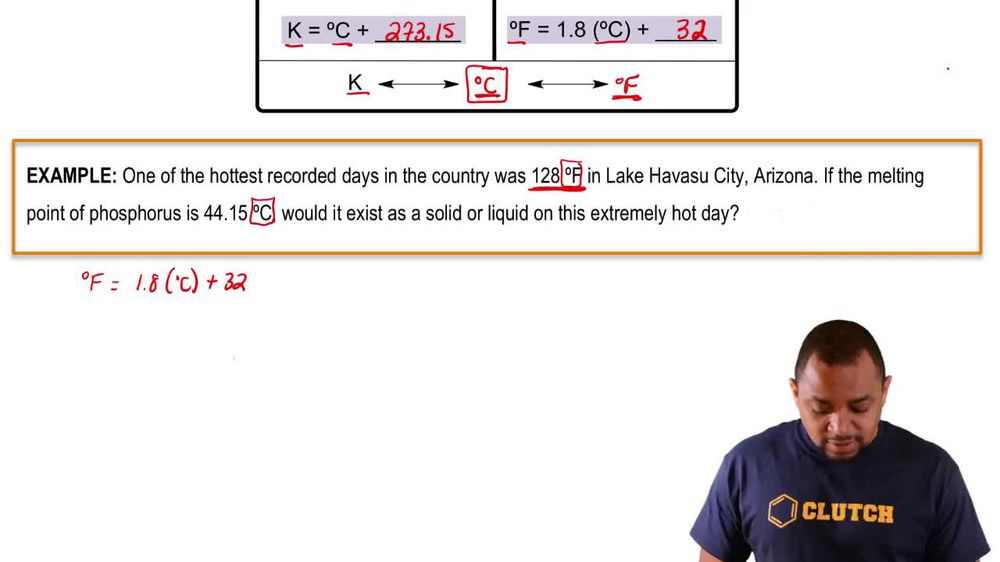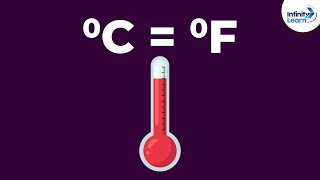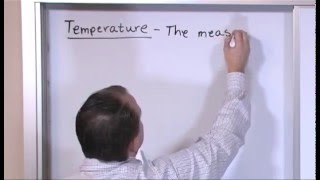Start typing, then use the up and down arrows to select an option from the list.
1. 1. Intro to General Chemistry2. Temperature
Problem

# The element gallium (Ga) has the second-largest liquid range of any element, melting at 29.78 °C and boiling at 2204 °C at atmospheric pressure. (b) Assume that you construct a thermometer using gallium as the fluid instead of mercury and that you define the melting point of gallium as 0 °G and the boiling point of gallium as 1000 °G. What is the melting point of sodium chloride (801 °C) on the gallium scale?

Relevant Solution3m
Play a video:
Hello everyone today. We are being given the following problem, rubidium Is one of the elements that exists as liquid just above room temperature. Its melting point is 39.31°C and it's boiling point is 688°C, assuming that a thermometer was constructed using rubidium instead of mercury. The melting point of rubidium is designated as zero degrees rubidium, while the boiling point of rubidium is designated as 1000 rubidium Determining melting point of potassium fluoride, 858°C on the rubidium scale. So our first step here is to write out everything that we have. So we know that we have rubidium. I believe it is our B and we have our melting point and we have our bowling point. So our original melting point is 39 degrees 39.1 degrees Celsius. While original boiling point is 688 degrees Celsius. However, when we switch over to the new scale, we have zero degrees rubidium as well as 1000 degrees rubidium. And so we're ultimately trying to find the melting point of potassium fluoride. And to do that, we have to do the following equation. So we have to take our rubidium scale boiling point and we have to subtract it by the original by the melting point rubidium and then we have to divide that by our boiling point for rubidium and Celsius subtracted by you guessed it. The melting point of rubidium On the C scale. And so when we do that, we get 1.54 2° rubidium over°C. But this is not our final answer. Next We have to find degrees rubidium and we can do that by using 1.542 or what we just sold for times our degrees associates minus Our 31.31 or our original melting point in C. And so our last step ultimately find the melting point of potassium fluoride is using that formula that we just constructed here are degrees rubidium 1.542 times that degrees C subtracted by the original melting point. We're going to substitute that C for 858°C as given in the question stem. And when we solve this, we get 1,262 Degrees rubidium as our final answer that we have solved the problem overall, I hope this helped. And until next time.